Courses

# Test: Lines And Angles - 2

## 20 Questions MCQ Test Mathematics (Maths) Class 7 | Test: Lines And Angles - 2

Description
This mock test of Test: Lines And Angles - 2 for Class 7 helps you for every Class 7 entrance exam. This contains 20 Multiple Choice Questions for Class 7 Test: Lines And Angles - 2 (mcq) to study with solutions a complete question bank. The solved questions answers in this Test: Lines And Angles - 2 quiz give you a good mix of easy questions and tough questions. Class 7 students definitely take this Test: Lines And Angles - 2 exercise for a better result in the exam. You can find other Test: Lines And Angles - 2 extra questions, long questions & short questions for Class 7 on EduRev as well by searching above.
QUESTION: 1

### In a right angled triangle where angle A= 90° and AB=AC. What are the values of angle B.

Solution:

∵ In ∆ABC,
AB = AC
∴ ∠B = ∠C    ...(1)
| Angles opposite to equal sides of a triangle are equal
In ∆ABC,
∠A + ∠B + ∠C = 180°
| Sum of all the angles of a triangle is 180°
⇒ 90° + ∠B + ∠C = 180°
| ∵ ∠A = 90° (given)
⇒    ∠B + ∠C = 90°    ...(2)
From (1) and (2), we get
∠B = ∠C = 45°.

QUESTION: 2

Solution:
QUESTION: 3

### What is the supplement of 105°

Solution:

Supplementary angles sums up to 180° x + 105° = 180° x=180° - 105° = 75°

QUESTION: 4

What is the measure of the complement of 45°?

Solution:

Complementary angle sums up to 90°. So x+ 45° = 90°
x = 90° - 45° = 45°

QUESTION: 5

What is the measure of the complement of 65°?

Solution:
QUESTION: 6

What is the measure of the complement of 41°?

Solution:

Let the angle be x°
then,complement°=(90-x)°

A/Q,
x=(90-41)
x=90-41
x=49

QUESTION: 7

What is the measure of the supplement of 54°?

Solution:
QUESTION: 8

Identify which of the following pairs of angles are complementary.

Solution:
QUESTION: 9

Identify which of the following pairs of angles are supplementary.

Solution:
QUESTION: 10

Find the angle, which is equal to its complement.

Solution:

Let one of the two equal complementary angles be x.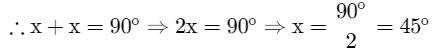Thus, 45is equal to its complement.

QUESTION: 11

Lines l║m, t is transversal then ∠ x ?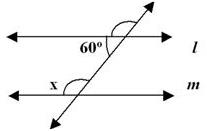Solution:
QUESTION: 12

Find the angle, which is equal to its supplement.

Solution:

Supplementary angles sums up to 180° since 90° + 90° = 180°, 90° is supplementary to itself

QUESTION: 13

Lines l║m, t is transversal then ∠ x = ?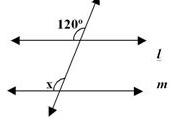Solution:

120 and x are corresponding angles and corresponding angles are equal so x = 120°

QUESTION: 14

Lines l║m, t is transversal then ∠ z = ?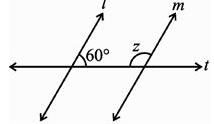Solution:

The angles are co-interior so x+60 = 180
x=120°

QUESTION: 15

Lines l║m, t is transversal then ∠ x = ?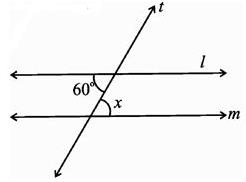Solution:
QUESTION: 16

ABC is a right angled triangle in which ∠A = 90° and AB = AC. Find ∠B and ∠C.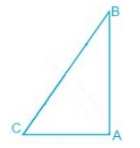Solution:

Given,
∠A = 90° and AB = AC
A/q,
AB = AC
⇒ ∠B = ∠C (Angles opposite to the equal sides are equal.)
Now,
∠A + ∠B + ∠C = 180° (Sum of the interior angles of the triangle.)
⇒ 90° + 2∠B = 180°
⇒ 2∠B = 90°
⇒ ∠B = 45°
Thus, ∠B = ∠C = 45°

QUESTION: 17

The measures of an angle supplement to the angle of 70° is ______.

Solution:
QUESTION: 18

Find the angle whose measure is five times its complement

Solution:

This will be the equation x=5(90-x)
solution= x=5(90-x)
x+5x=90
6x=90
6x/6=90/6   {divide by 6 to both the side to x}
x=15

ans= The angle is 15 degree and its compliment angle is 75 degreeQUESTION: 19

Find x if I║m.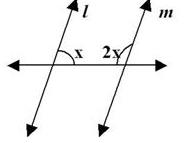Solution:
QUESTION: 20

Find the value of x if I║m.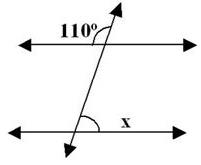Solution: